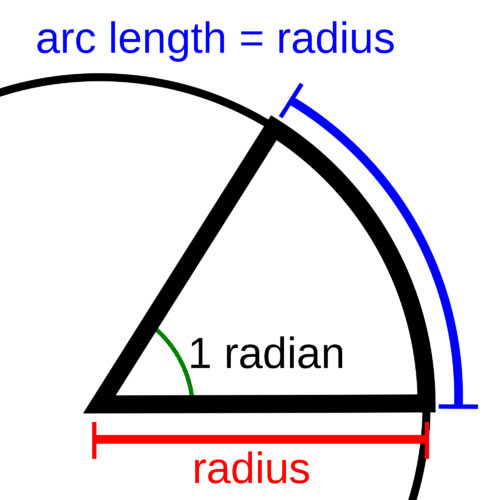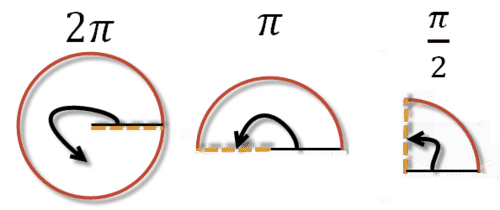# 4.1: Angles in Radians and Degrees

•• Contributed by CK12
• CK12

Most people are familiar with measuring angles in degrees. It is easy to picture angles like $$30^{\circ}, 45^{\circ}$$ or $$90^{\circ}$$ and the fact that $$360^{\circ}$$ makes up an entire circle. Over 2000 years ago the Babylonians used a base 60 number system and divided up a circle into 360 equal parts. This became the standard and it is how most people think of angles today.

However, there are many units with which to measure angles. For example, the gradian was invented along with the metric system and it divides a circle into 400 equal parts. The sizes of these different units are very arbitrary.

A radian is a unit of measuring angles that is based on the properties of circles. This makes it more meaningful than gradians or degrees. How many radians make up a circle?

radian is defined to be the central angle where the subtended arc length is the same length as the radius.Another way to think about radians is through the circumference of a circle. The circumference of a circle with radius $$r$$ is $$2 \pi r$$. Just over six radii (exactly $$2 \pi$$ radii) would stretch around any circle.

To define a radian in terms of degrees, equate a circle measured in degrees to a circle measured in radians.

360 degrees $$=2 \pi$$ radians, so $$\frac{180}{\pi}$$ degrees $$=1$$ radian

Alternatively, 360 degrees $$=2 \pi$$ radians, so 1 degree $$=\frac{\pi}{180}$$ radians

The conversion factor to convert degrees to radians is: $$\frac{\pi}{180^{\circ}}$$

The conversion factor to convert radians to degrees is: $$\frac{180^{\circ}}{\pi}$$

If an angle has no units, it is assumed to be in radians.

If you were to convert $$150^{\circ}$$ into radians, you would multiply $$150^{\circ}$$ by the correct conversion factor. You would get:

$$150^{\circ} \cdot \frac{\pi}{180^{\circ}}=\frac{15 \pi}{18}=\frac{5 \pi}{6}$$ radians

You can check your work by making sure the degree units appear on both the numerator and denominator.

If you were to convert $$\frac{\pi}{6}$$ radians into degrees, you would multiply $$\frac{\pi}{6}$$ by the correct conversion factor. You would get $$\frac{\pi}{6} \cdot \frac{180^{\circ}}{\pi}=\frac{180^{\circ}}{6}=30^{\circ}$$

Notice $$\pi$$ appears in both the numerator and denominator and $$\frac{\pi}{\pi}=1$$.

### Examples

Example 1

Earlier, you were asked how many radians make up a circle. Exactly $$2 \pi$$ radians describe a circular arc. This is because $$2 \pi$$ radii wrap around the circumference of any circle.

Example 2

Convert $$(6 \pi)^{\circ}$$ into radians.

Don't be fooled just because this has $$\pi$$. This number is about $$19^{\circ}$$

$$(6 \pi)^{\circ} \cdot \frac{\pi}{180^{\circ}}=\frac{6 \pi^{2}}{180}=\frac{\pi^{2}}{3}$$

It is very unusual to ever have a $$\pi^{2}$$ term, but it can happen.

Example 3

Convert $$\frac{5 \pi}{6}$$ into degrees.

$$\frac{5 \pi}{6} \cdot \frac{180^{\circ}}{\pi}=\frac{5 \cdot 30^{\circ}}{1}=150^{\circ}$$

Example 4

Convert $$210^{\circ}$$ into radians.

$$210^{\circ} \cdot \frac{\pi}{180^{\circ}}=\frac{7 \cdot 30 \cdot \pi}{6 \cdot 30}=\frac{7 \pi}{6}$$

Example 5

Draw a $$\frac{\pi}{2}$$ angle by first drawing a $$2 \pi$$ angle, halving it and halving the result. Recall that $$\frac{\pi}{2}=90^{\circ}$$.Review

Find the radian measure of each angle.

1. $$120^{\circ}$$

2. $$300^{\circ}$$

3. $$90^{\circ}$$

4. $$330^{\circ}$$

5. $$270^{\circ}$$

6. $$45^{\circ}$$

7. $$(5 \pi)^{\circ}$$

Find the degree measure of each angle.

8. $$\frac{7 \pi}{6}$$

9. $$\frac{5 \pi}{4}$$

10. $$\frac{3 \pi}{2}$$

11. $$\frac{5 \pi}{3}$$

12. $$\pi$$

13. $$\frac{\pi}{6}$$

14. 3

15. Explain why if you are given an angle in degrees and you multiply it by $$\frac{\pi}{180}$$ you will get the same angle in radians.